# 童晶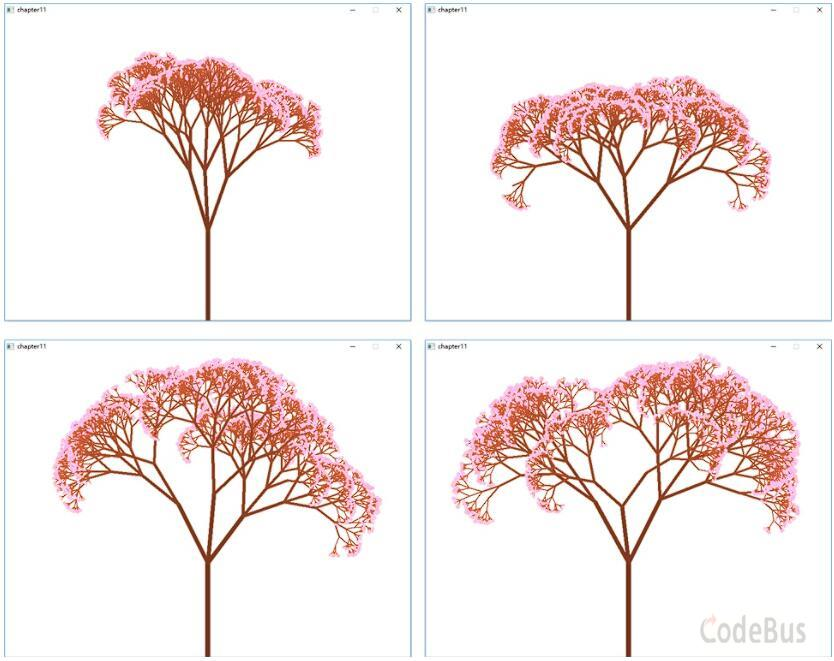``````#include <graphics.h>
#include <conio.h>
#include <stdio.h>
#include <math.h>
#include <time.h>
#define  PI 3.1415926
#define  WIDTH 800   // 画面宽度
#define  HEIGHT 600  // 画面高度度

float offsetAngle = PI/6; // ``````
...``````#include <graphics.h>
#include <conio.h>
#include <stdio.h>
#include <time.h>
#include <stdlib.h>

# define BlockSize 4``````
...``````#include <graphics.h>
#include <conio.h>
#include <stdio.h>
#define B_SIZE 60 // 方块大小
#define B_NUM 8 // 方块个数，一共8*8个方块

struct Player // 结构体，用于记录玩家位置
{
int i;
int j;
};
Player player; // 玩家全局变量

enum E``````
...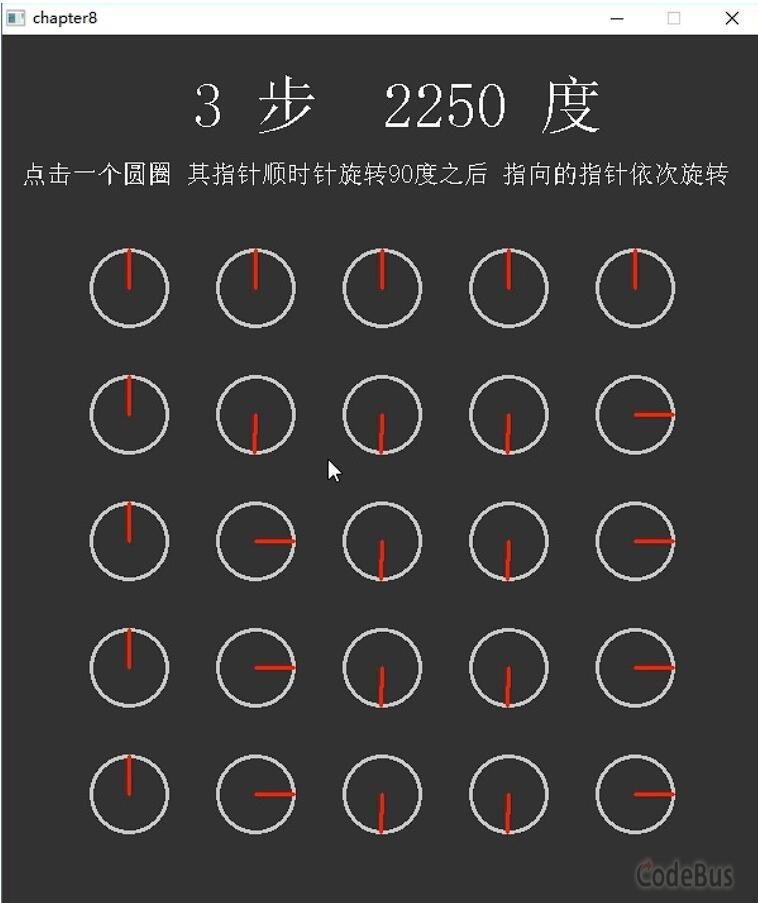``````#include <graphics.h>
#include <conio.h>
#include <math.h>
#define PI 3.14159 // PI宏定义

struct Round // 定义结构体，用来表示带角度指示的小圆圈
{
float x,y; // 小圆的圆心坐标
float r; // 小圆半径
``````
...``````#include <graphics.h>
#include <conio.h>
#include <stdio.h>
#define BLOCK_SIZE 20 // 每个小格子的长宽大小
#define HEIGHT 30 // 高度上一共30个小格子
#define WIDTH 40 //  宽度上一共40个小格子

// 全局变量定义
int Blocks[HEIGH``````
...``````#include <graphics.h>
#include <conio.h>
#include <stdio.h>
#include <time.h>
#include <math.h>

// 求解两个点之间的距离
float Dist2Points(float x1,float y1,float x2,float y2)
{
float result;
resul``````
...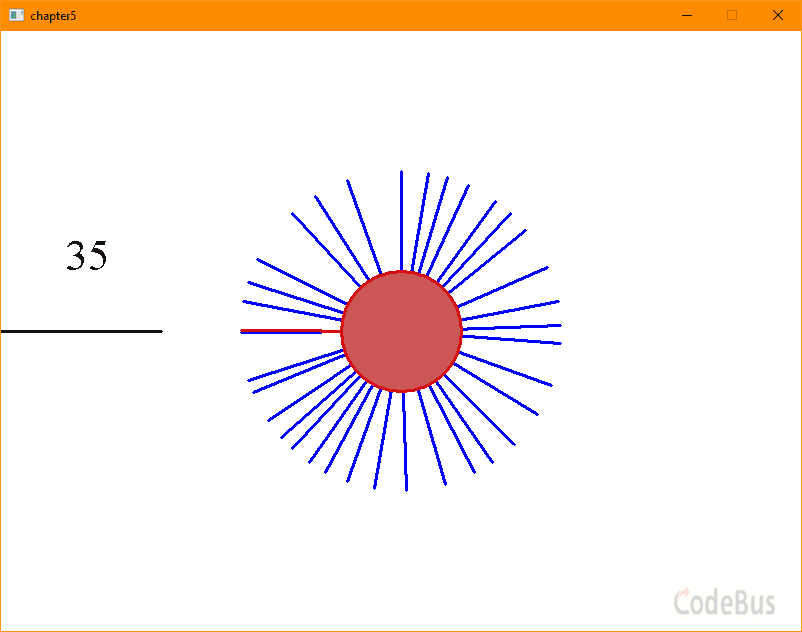``````#include <graphics.h>
#include <conio.h>
#include <stdio.h>
#include <math.h>
int main()
{
const float Pi = 3.1415926; // Pi常量
int width = 800; // 画面宽度
int height = 600; // 画面高度
initgra``````
...``````#include <graphics.h>
#include <conio.h>
#include <stdio.h>
#include <time.h>
int main()
{
float Pi = 3.14159;  // 圆周率Pi
initgraph(800,600); // 打开一个窗口
setbkcolor(RGB(128,128,128)); // 设置背景颜色为灰色
cleardevice();  // 以背``````
...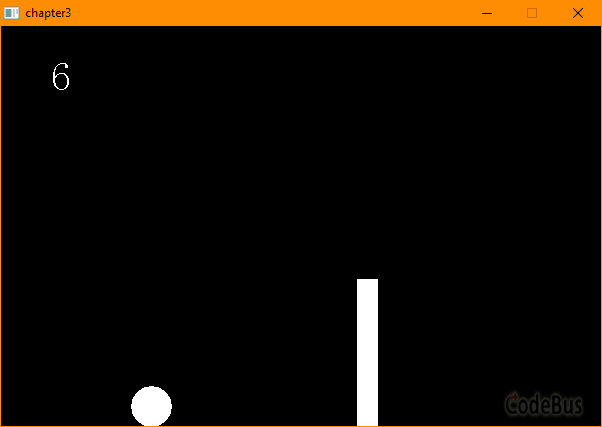``````#include <graphics.h>
#include <conio.h>
#include <stdio.h>
int main()
{
float width,height,gravity; // 游戏画面大小、重力加速度
float rect_left_x,rect_top_y,rect_width,rect_height,re``````
...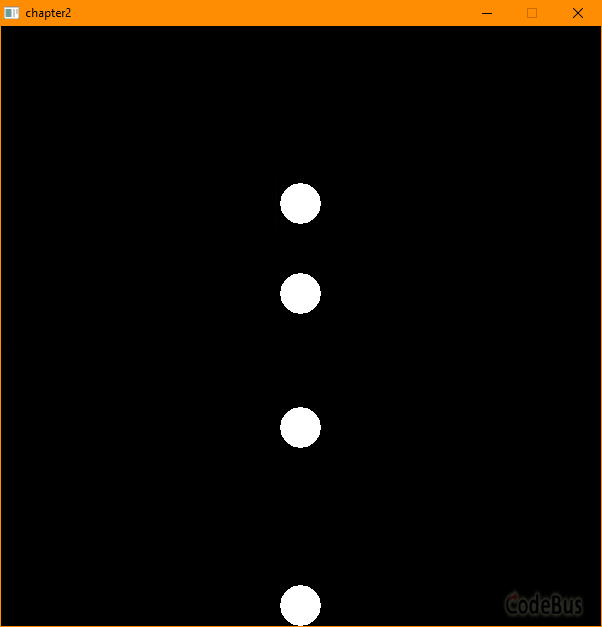# 源码

``````#include <graphics.h>
#include <conio.h>

int main()
{
float y = 100;				// 小球的 y 坐标
float vy = 0;				// 小球 y 方向速度
float g = 0.5;				// 小球加速度，y 方向
initgraph(600, 600);		// 初始游戏窗口画面，``````
...